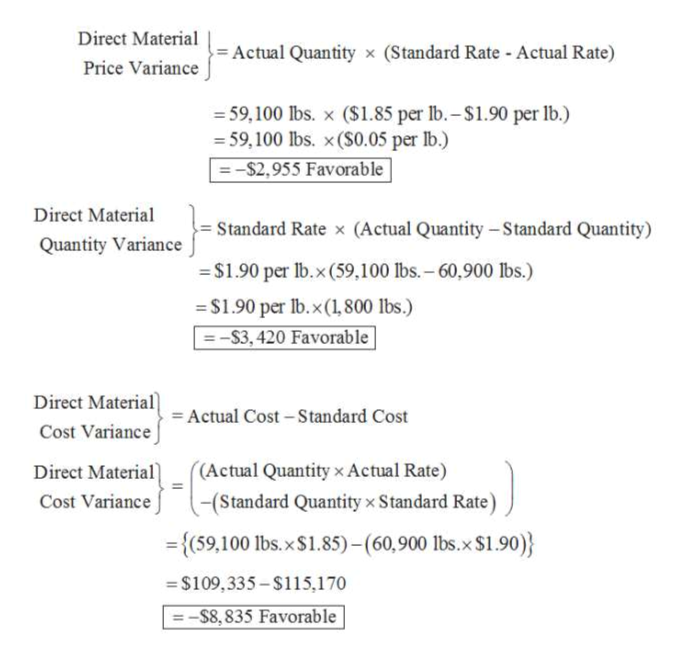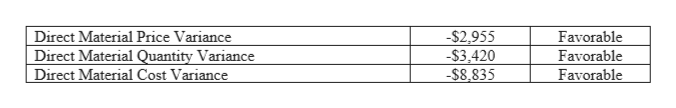# Direct Materials VariancesThe following data relate to the direct materials cost for the production of 2,300 automobile tires:Actual:59,100 lbs. at \$1.85 per lb. Standard:60,900 lbs. at \$1.90 per lb. a.  Determine the direct materials price variance, direct materials quantity variance, and total direct materials cost variance. Enter a favorable variance as a negative number using a minus sign and an unfavorable variance as a positive number.Direct Materials Price Variance\$ Direct Materials Quantity Variance\$ Total Direct Materials Cost Variance\$ b.  The direct materials price variance should normally be reported to the  . When lower amounts of direct materials are used because of production efficiencies, the variance would be reported to the  . When the favorable use of raw materials is caused by the purchase of higher-quality raw materials, the variance should be reported to the  .

Question
2 views

Direct Materials Variances

The following data relate to the direct materials cost for the production of 2,300 automobile tires:

 Actual: 59,100 lbs. at \$1.85 per lb. Standard: 60,900 lbs. at \$1.90 per lb.

a.  Determine the direct materials price variance, direct materials quantity variance, and total direct materials cost variance. Enter a favorable variance as a negative number using a minus sign and an unfavorable variance as a positive number.

 Direct Materials Price Variance \$ Direct Materials Quantity Variance \$ Total Direct Materials Cost Variance \$

b.  The direct materials price variance should normally be reported to the  . When lower amounts of direct materials are used because of production efficiencies, the variance would be reported to the  . When the favorable use of raw materials is caused by the purchase of higher-quality raw materials, the variance should be reported to the  .

check_circle

Step 1

a.  Calculation of direct material price variance, direct material quantity variance and direct material cost variance:help_outlineImage TranscriptioncloseDirect Material = Actual Quantity x (Standard Rate - Actual Rate) Price Variance = 59,100 lbs. x (\$1.85 per lb.–\$1.90 per lb.) = 59,100 lbs. x (S0.05 per lb.) =-\$2,955 Favorable Direct Material = Standard Rate x (Actual Quantity -Standard Quantity) Quantity Variance = \$1.90 per lb.x(59,100 lbs. – 60,900 lbs.) = \$1.90 per lb.x(1,800 lbs.) = -S3, 420 Favorable Direct Material = Actual Cost – Standard Cost Cost Variance ((Actual Quantity x Actual Rate) Direct Material) (Standard Quantity x Standard Rate) Cost Variance = {(59,100 lbs.x\$1.85) -(60,900 lbs.x \$1.90)} = \$109,335 – \$115,170 –S8, 835 Favorable fullscreen
Step 2

Therefore...help_outlineImage TranscriptioncloseDirect Material Price Variance Direct Material Quantity Variance Direct Material Cost Variance -\$2,955 -\$3,420 -\$8.835 Favorable Favorable Favorable fullscreen

### Want to see the full answer?

See Solution

#### Want to see this answer and more?

Solutions are written by subject experts who are available 24/7. Questions are typically answered within 1 hour.*

See Solution
*Response times may vary by subject and question.
Tagged in